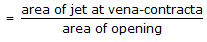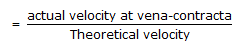# Chemical Engineering - Fluid Mechanics

21.

In case of isentropic flow, the speed of sound in an ideal gas is proportional to (where, T = absolute temperature )

 A. 1/T B. 1/T C. T D. T

Explanation:

No answer description available for this question. Let us discuss.

22.

Applying a pressure drop across a capillary results in a volumetric flow rate 'Q' under laminar flow conditions. The flow rate for the same pressure drop, in a capillary of the same length but half the radius is

 A. Q/2 B. Q/4 C. Q/8 D. Q/16

Explanation:

No answer description available for this question. Let us discuss.

23.

Cd, Cc and Cv are related (for flow through an orifice) as (where, Cd = discharge co-efficient
Cc = co-efficient of contractionCv = co-efficient of velocity)

 A. Cd = Cc/Cv B. Cd = Cc.Cv C. Cd = Cv/cc D. none of these

Explanation:

No answer description available for this question. Let us discuss.

24.

In an incompressible fluid, the density is

 A. greatly affected by moderate changes in pressure. B. greatly affected only by moderate changes in temperature. C. not affected with moderate change in temperature & pressure. D. sensible to changes in both temperature & pressure.

Explanation:

No answer description available for this question. Let us discuss.

25.

Check valves are used

 A. at high pressure. B. in bends. C. for controlling water flow. D. for unidirectional flow.